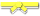# Search Results

Searching for: String

## Individual Exercises

0 / 50

Write a function in Java that implements the following logic: Given a string name, e.g. "Bob", return a greeting of the form "Hello...0 / 50

Write a function in Java that implements the following logic: Given a string, return true if it ends in "ly".0 / 50

Write a function in Java that implements the following logic: Given a string, if the string begins with "red" or "blue" return that color...0 / 50

Write a function in Java that implements the following logic: The web is built with HTML strings like "<i>Yay</i>" which...0 / 50

Write a function in Java that implements the following logic: Given 2 strings, a and b, return a string of the form short+long+short,...0 / 50

Write a function in Java that implements the following logic: Given a string, return a string made of its first 2 chars. If the string...0 / 50

Write a function in Java that implements the following logic: Given a string, return a string of length 1 from its front, unless `front` is...0 / 50

Write a function in Java that implements the following logic: Given a string and an int `n`, return a string made of `n` repetitions of the...0 / 50

Write a function in Java that implements the following logic: Given a string `str` and a non-empty `word`, return a version of the original...0 / 50

Write a function in Java that implements the following logic: Given two strings, `a` and `b`, create a bigger string made of the first...0 / 50

Given a string `str`, find all places where a three-letter combination starting with "z" and ending with "p" occurs. Return a string where...0 / 50

Write a function in Java that implements the following logic: Given a string `str` and a non-empty `word`, return a string made of each...0 / 50

Write a function in Java that counts the number of times the string "code" appears anywhere in the given string `str`, except that we'll...0 / 50

Given two strings, return true if either of the strings appears at the very end of the other string, ignoring upper/lower case...0 / 50

Write a function in Java that returns true if the given string `str` contains an occurrence of the substring "xyz" where "xyz" is not...0 / 50

We'll say that a string is xy-balanced if for all the 'x' characterss in the string, there exists a 'y' character somewhere later in the...0 / 50

A sandwich is two pieces of bread with something in between. Write a Java method that takes in a string `str` and returns the string that...0 / 50

Given a string, if the same 2-character substring appears at both its beginning and end, return the contents of the string without the...0 / 50

Given a string of even length, return the first half. So the string "WooHoo" yields "Woo".0 / 50

Given a string, does "xyz" appear in the middle of the string? To define middle, we'll say that the number of chars to the left and right...0 / 50

Given a string `str` and an `int n`, return a string made of the first `n` characters of the string, followed by the first `n - 1` characters of...0 / 30

Given a string, return `true` if "bad" appears starting at index 0 or 1 in the string, such as with "badxxx" or "xbadxx" but not "xxbadxx"....0 / 50

Return a version of the given string, where for every star (*) in the string the star and the character immediately to its left and the...0 / 30

Given an `out` string of length 4, such as "<<>>", and a `word`, return a new string where the `word` is in the middle of the `out`...0 / 30

Given a string, return a new string made of 3 copies of the last 2 chars of the original string. The string's length will be at least 2.

...